1. Standard Electrode Potentials (Redox Potentials)

Background

The differing reactivities of metals

When metals react, they give away electrons and form positive ions. This particular topic sets about comparing the ease with which a metal does this to form hydrated ions in solution - for example, Mg2+(aq) or Cu2+(aq).

We might want to compare the ease with which these two changes take place: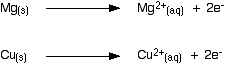Everybody who has done chemistry for more than a few months knows that magnesium is more reactive than copper. The first reaction happens much more readily than the second one. What this topic does is to try to express this with some numbers.

Looking at this from an equilibrium point of view

Suppose you have a piece of magnesium in a beaker of water. There will be some tendency for the magnesium atoms to shed electrons and go into solution as magnesium ions. The electrons will be left behind on the magnesium.In a very short time, there will be a build-up of electrons on the magnesium, and it will be surrounded in the solution by a layer of positive ions. These will tend to stay close because they are attracted to the negative charge on the piece of metal.

Some of them will be attracted enough that they will reclaim their electrons and stick back on to the piece of metal.A dynamic equilibrium will be established when the rate at which ions are leaving the surface is exactly equal to the rate at which they are joining it again. At that point there will be a constant negative charge on the magnesium, and a constant number of magnesium ions present in the solution around it.

Simplifying the diagram to get rid of the "bites" out of the magnesium, you would be left with a situation like this: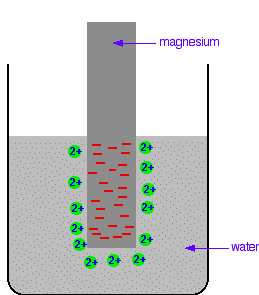Don't forget that this is just a snapshot of a dynamic equilibrium. Ions are continually leaving and rejoining the surface.

How would this be different if you used a piece of copper instead of a piece of magnesium?

Copper is less reactive and so forms its ions less readily. Any ions which do break away are more likely to reclaim their electrons and stick back on to the metal again. You will still reach an equilibrium position, but there will be less charge on the metal, and fewer ions in solution.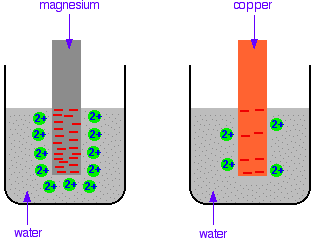If we write the two reactions as equilibria, then what we are doing is comparing the two positions of equilibrium.

The position of the magnesium equilibrium . . .. . . lies further to the left than that of the copper equilibrium.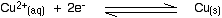Notice the way that the two equilibria are written. By convention, all these equilibria are written with the electrons on the left-hand side of the equation. If you stick with this convention without fail, you will find that it makes the rest of this topic much easier to visualise.

Everything else concerning electrode potentials is simply an attempt to attach some numbers to these differing positions of equilibrium.

In principle, that is quite easy to do. In the magnesium case, there is a lot of difference between the negativeness of the metal and the positiveness of the solution around it. In the copper case, the difference is much less.

This potential difference could be recorded as a voltage - the bigger the difference between the positiveness and the negativeness, the bigger the voltage. Unfortunately, that voltage is impossible to measure!

It would be easy to connect a voltmeter to the piece of metal, but how would you make a connection to the solution? By putting a probe into the solution near the metal? No - it wouldn't work!

Any probe you put in is going to have a similar sort of equilibrium happening around it. The best you could measure would be some sort of combination of the effects at the probe and the piece of metal you are testing.

Understanding the ideas behind a reference electrode

Suppose you had an optical device for measuring heights some distance away, and wanted to use it to find out how tall a particular person was. Unfortunately, you can't see their feet because they are standing in long grass.Although you can't measure their absolute height, what you can do is to measure their height relative to the convenient post. Suppose that in this case, the person turned out to be 15 cm taller than the post.

You could repeat this for a range of people . . .. . . and come up with a set of results like this:

person height relative to post (cm)
C +20
A +15
B -15

Although you don't know any of their absolute heights, you can usefully rank them in order, and do some very simple sums to work out exactly how much taller one is than another. For example, C is 5 cm taller than A.

This turns out to be exactly what we need to do with the equilibria we started talking about. We don't actually need to know the absolute position of any of these equilibria. Going back to the magnesium and copper equilibria:All we need to know is that the magnesium equilibrium lies further to the left than the copper one. We need to know that magnesium sheds electrons and forms ions more readily than copper does.

That means that we don't need to be able to measure the absolute voltage between the metal and the solution. It is enough to compare the voltage with a standardised system called a reference electrode.

The system used is called a standard hydrogen electrode.

Measuring standard electrode potentials (standard redox potentials)

The standard hydrogen electrode

The standard hydrogen electrode looks like this:What is happening?

As the hydrogen gas flows over the porous platinum, an equilibrium is set up between hydrogen molecules and hydrogen ions in solution. The reaction is catalysed by the platinum.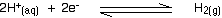This is the equilibrium that we are going to compare all the others with.

Standard conditions

The position of any equilibrium can be changed by changing conditions. That means that the conditions must be standardised so that you can make fair comparisons.

The hydrogen pressure is 1 bar (100 kPa). (You may find 1 atmosphere quoted in older sources.) The temperature is 298 K (25°C).

The concentration of the hydrogen ions in solution is also important. Changing concentrations is one of the ways of changing the position of an equilibrium. Throughout this topic, all ion concentrations are taken as being 1 mol dm-3.

Using the standard hydrogen electrode

The standard hydrogen electrode is attached to the electrode system you are investigating - for example, a piece of magnesium in a solution containing magnesium ions.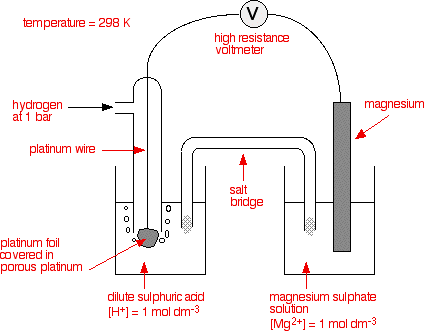Cells and half cells

The whole of this set-up is described as a cell. It is a simple system which generates a voltage. Each of the two beakers and their contents are described as half cells.

The salt bridge

The salt bridge is included to complete the electrical circuit but without introducing any more bits of metal into the system. It is just a glass tube filled with an electrolyte like potassium nitrate solution. The ends are "stoppered" by bits of cotton wool. This stops too much mixing of the contents of the salt bridge with the contents of the two beakers.

The electrolyte in the salt bridge is chosen so that it doesn't react with the contents of either beaker.

What happens?

These two equilibria are set up on the two electrodes (the magnesium and the porous platinum):Magnesium has a much greater tendency to form its ions than hydrogen does. The position of the magnesium equilibrium will be well to the left of that of the hydrogen equilibrium.

That means that there will be a much greater build-up of electrons on the piece of magnesium than on the platinum. Stripping all the rest of the diagram out, apart from the essential bits: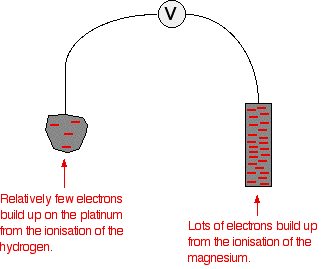There is a major difference between the charge on the two electrodes - a potential difference which can be measured with a voltmeter. The voltage measured would be 2.37 volts and the voltmeter would show the magnesium as the negative electrode and the hydrogen electrode as being positive.

This sometimes confuses people! Obviously, the platinum in the hydrogen electrode isn't positive in real terms - there is a slight excess of electrons built up on it. But voltmeters don't deal in absolute terms - they simply measure a difference.

The magnesium has the greater amount of negativeness - the voltmeter records that as negative. The platinum of the hydrogen electrode isn't as negative - it is relatively more positive. The voltmeter records it as positive.

Throughout the whole of this redox potential work, you have to think in relative terms. For example, +0.4 is relatively more negative than +1.2. Or, another example, -0.3 is relatively more positive than -0.9.

What if you replace the magnesium half cell by a copper one?

This means replacing the magnesium half cell by one with a piece of copper suspended in a solution containing Cu2+ ions with a concentration of 1 mol dm-3. You would probably choose to use copper(II) sulphate solution.

Copper forms its ions less readily than hydrogen does. Of the two equilibria . . .. . . the hydrogen one lies further to the left. That means that there will be less build-up of electrons on the copper than there is on the platinum of the hydrogen electrode.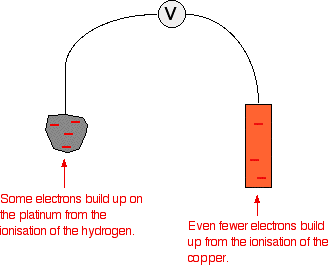There is less difference between the electrical charges on the two electrodes, so the voltage measured will be less. This time it is only 0.34 volts.

The other major change is that this time the copper is the more positive (less negative) electrode. The voltmeter will show the hydrogen electrode as the negative one and the copper electrode as positive.

The voltmeter

You may have noticed that the voltmeter was described further up the page as having a "high resistance". Ideally, it wants to have an infinitely high resistance.

This is to avoid any flow of current through the circuit. If there was a low resistance in the circuit, electrons would flow from where there are a lot of them (around the magnesium, for example) to where there are less (on the hydrogen electrode).

If any current flows, the voltage measured drops. In order to make proper comparisons, it is important to measure the maximum possible voltage in any situation. This is called the electromotive force or emf.

The emf of a cell measured under standard conditions is given the symbol E°cell.

Cell conventions

A quick way of drawing a cell

Drawing a full diagram to represent a cell takes too long. Instead, the cell in which a magnesium electrode is coupled to a hydrogen electrode is represented like this:You will often find variants on the way the hydrogen electrode is represented, such as: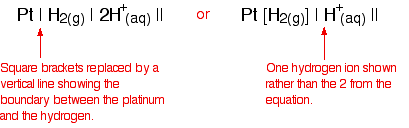Attaching a sign to the cell voltage

The convention is that you show the sign of the right-hand electrode (as you have drawn it) when you quote the E°cell value. For example: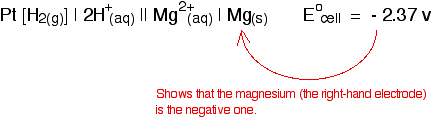In the copper case: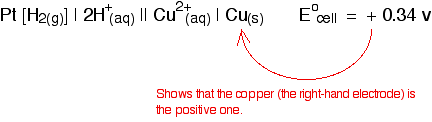Defining standard electrode potential (standard redox potential)

The values that we have just quoted for the two cells are actually the standard electrode potentials of the Mg2+ / Mg and Cu2+ / Cu systems.

The emf measured when a metal / metal ion electrode is coupled to a hydrogen electrode under standard conditions is known as the standard electrode potential of that metal / metal ion combination.

By convention, the hydrogen electrode is always written as the left-hand electrode of the cell. That means that the sign of the voltage quoted always gives you the sign of the metal electrode.

Standard electrode potential is given the symbol .

Summarizing what standard electrode potentials tell you

Remember that the standard electrode potential of a metal / metal ion combination is the emf measured when that metal / metal ion electrode is coupled to a hydrogen electrode under standard conditions.

What you are doing is comparing the position of the metal / metal ion equilibrium with the equilibrium involving hydrogen.

Here are a few typical standard electrode potentials:

metal / metal ion combination E° (volts)
Mg2+ / Mg -2.37
Zn2+ / Zn -0.76
Cu2+ / Cu +0.34
Ag+ / Ag +0.80

Remember that each of these is comparing the position of the metal / metal ion equilibrium with the equilibrium involving hydrogen.

Here are the five equilibria (including the hydrogen one):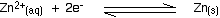If you compare these with the E° values, you can see that the ones whose positions of equilibrium lie furthest to the left have the most negative E° values. That is because they form ions more readily - and leave more electrons behind on the metal, making it more negative.

Those which don't shed electrons as readily have positions of equilibrium further to the right. Their E° values get progressively more positive.

A final summary

E° values give you a way of comparing the positions of equilibrium when these elements lose electrons to form ions in solution.

• The more negative the E° value, the further the equilibrium lies to the left - the more readily the element loses electrons and forms ions.

• The more positive (or less negative) the E° value, the further the equilibrium lies to the right - the less readily the element loses electrons and forms ions.